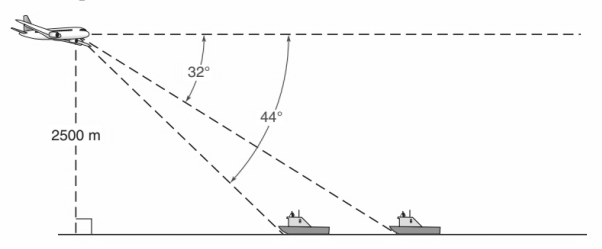Chapter 11.CR, Problem 30CRElementary Geometry For College St...

7th Edition
Alexander + 2 others
ISBN: 9781337614085

Solutions

Chapter
SectionElementary Geometry For College St...

7th Edition
Alexander + 2 others
ISBN: 9781337614085
Textbook Problem

In Review Exercises 21 to 30, use the drawings, where provided, to solve each problem, Angle measures should be found to the nearest degree; lengths should be found to the nearest tenth of a unit.An observer in a plane 2500 m high sights two ships below. The angle of depression to one ship is 32 ° , and the angle of depression to the other ship is 44 ° . How far apart are the ships?To determine

To find:

The distance between the ships. An observer in a plane 2500 m high sights two ships below, the angle of depression to one ship is 32° and for other ship. 44°.

Explanation

Procedure used:

In any right triangle ABC with mA=θ we have the following ratios.

sinθ=OppositeHypotenuse

Calculation:

Given:

Height of the plane =2500 m.

Angle of depression of the ships are 32° and 44°.

Let us structure the given figure as a right triangle as follows.

From the above figure we have

CD=2500 m,

mZDA=32°, and

mZDB=44°

Let ‘x’ be the distance between the ships.

AB=x

BC=y (say), and

CA=BC+AB

CA=x+y`

Also by the property that the alternate interior angles are equal we have

mZDA=mDAC=32° and

mZDB=mDBC=44°

In the above figure we have two right angle triangle BCD and ACD.

Take the BCD and apply the ratio tanθ=Oppositeadjacent

tan44°=CDBC

0

Still sussing out bartleby?

Check out a sample textbook solution.

See a sample solution

The Solution to Your Study Problems

Bartleby provides explanations to thousands of textbook problems written by our experts, many with advanced degrees!

Get Started

If 28f(x)dx=7.3 and 24f(x)dx=5.9, find 48f(x)dx.

Single Variable Calculus: Early Transcendentals, Volume I

Limits at Infinity Find the limit. 13. limxx41x2+x3

Precalculus: Mathematics for Calculus (Standalone Book)

True or False: is a convergent series.

Study Guide for Stewart's Multivariable Calculus, 8th

An estimate of 13x4 using Simpsons Rule with n = 4 gives: a) 2425 b) 1483 c) 1523 d) 2445

Study Guide for Stewart's Single Variable Calculus: Early Transcendentals, 8th